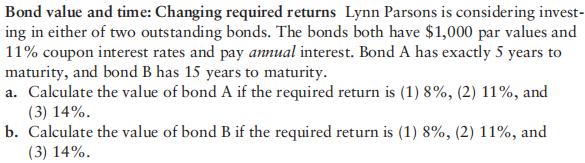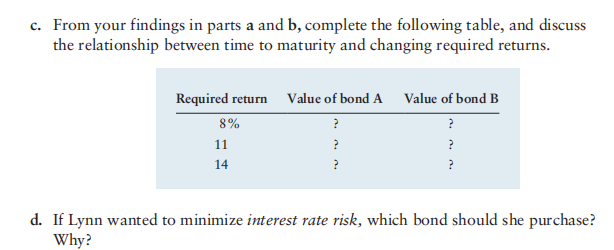# Question Bond value and time: Changing required returns Lynn Parsons is considering investing in either of two outstanding bonds. The bonds both have $$\ 1,000$$ par values and $$11 \%$$ coupon interest rates and pay annual interest. Bond A has exactly 5 years to maturity, and bond B has 15 years to maturity. a. Calculate the value of bond $$\mathrm{A}$$ if the required return is (1) $$8 \%$$, (2) $$11 \%$$, and (3) $$14 \%$$. b. Calculate the value of bond $$\mathrm{B}$$ if the required return is (1) $$8 \%,(2) 11 \%$$, and (3) $$14 \%$$.>c. From your findings in parts a and $$\mathbf{b}$$, complete the following table, and discuss the relationship between time to maturity and changing required returns. \begin{tabular}{ccc} Required return & Value of bond A & Value of bond B \\ \hline $$8 \%$$ & $$?$$ & $$?$$ \\ 11 & $$?$$ & $$?$$ \\ 14 & $$?$$ & $$?$$ \end{tabular} d. If Lynn wanted to minimize interest rate risk, which bond should she purchase? Why?Bond value and time: Changing required returns Lynn Parsons is considering investing in either of two outstanding bonds. The bonds both have $$\ 1,000$$ par values and $$11 \%$$ coupon interest rates and pay annual interest. Bond A has exactly 5 years to maturity, and bond B has 15 years to maturity.
a. Calculate the value of bond $$\mathrm{A}$$ if the required return is (1) $$8 \%$$, (2) $$11 \%$$, and (3) $$14 \%$$.
b. Calculate the value of bond $$\mathrm{B}$$ if the required return is (1) $$8 \%,(2) 11 \%$$, and (3) $$14 \%$$.>c. From your findings in parts a and $$\mathbf{b}$$, complete the following table, and discuss the relationship between time to maturity and changing required returns.
\begin{tabular}{ccc}
Required return & Value of bond A & Value of bond B \\
\hline $$8 \%$$ & $$?$$ & $$?$$ \\
11 & $$?$$ & $$?$$ \\
14 & $$?$$ & $$?$$
\end{tabular}
d. If Lynn wanted to minimize interest rate risk, which bond should she purchase? Why?Transcribed Image Text: Bond value and time: Changing required returns Lynn Parsons is considering investing in either of two outstanding bonds. The bonds both have $$\ 1,000$$ par values and $$11 \%$$ coupon interest rates and pay annual interest. Bond A has exactly 5 years to maturity, and bond B has 15 years to maturity. a. Calculate the value of bond $$\mathrm{A}$$ if the required return is (1) $$8 \%$$, (2) $$11 \%$$, and (3) $$14 \%$$. b. Calculate the value of bond $$\mathrm{B}$$ if the required return is (1) $$8 \%,(2) 11 \%$$, and (3) $$14 \%$$.>c. From your findings in parts a and $$\mathbf{b}$$, complete the following table, and discuss the relationship between time to maturity and changing required returns. \begin{tabular}{ccc} Required return & Value of bond A & Value of bond B \\ \hline $$8 \%$$ & $$?$$ & $$?$$ \\ 11 & $$?$$ & $$?$$ \\ 14 & $$?$$ & $$?$$ \end{tabular} d. If Lynn wanted to minimize interest rate risk, which bond should she purchase? Why?
More
Transcribed Image Text: Bond value and time: Changing required returns Lynn Parsons is considering investing in either of two outstanding bonds. The bonds both have $$\ 1,000$$ par values and $$11 \%$$ coupon interest rates and pay annual interest. Bond A has exactly 5 years to maturity, and bond B has 15 years to maturity. a. Calculate the value of bond $$\mathrm{A}$$ if the required return is (1) $$8 \%$$, (2) $$11 \%$$, and (3) $$14 \%$$. b. Calculate the value of bond $$\mathrm{B}$$ if the required return is (1) $$8 \%,(2) 11 \%$$, and (3) $$14 \%$$.>c. From your findings in parts a and $$\mathbf{b}$$, complete the following table, and discuss the relationship between time to maturity and changing required returns. \begin{tabular}{ccc} Required return & Value of bond A & Value of bond B \\ \hline $$8 \%$$ & $$?$$ & $$?$$ \\ 11 & $$?$$ & $$?$$ \\ 14 & $$?$$ & $$?$$ \end{tabular} d. If Lynn wanted to minimize interest rate risk, which bond should she purchase? Why?